Anúncio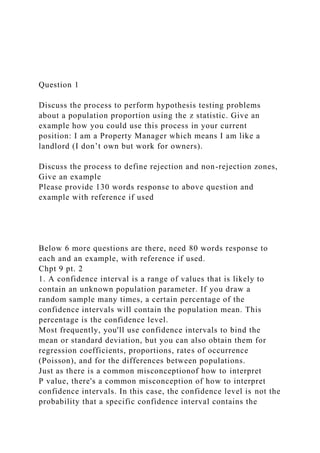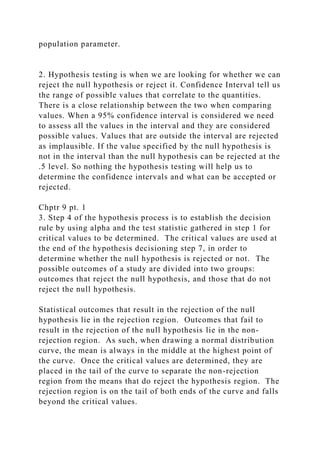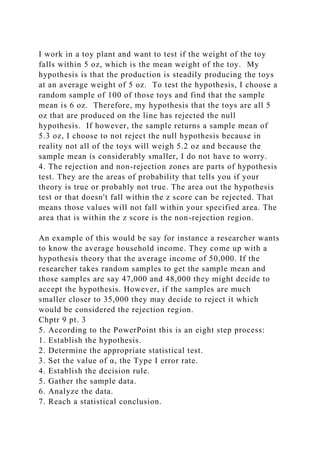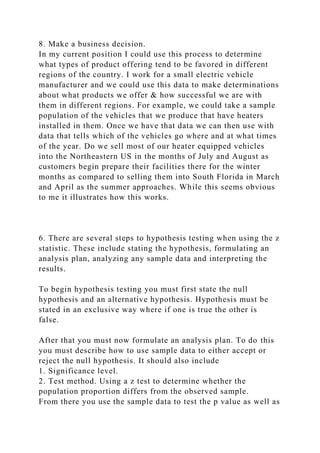Anúncio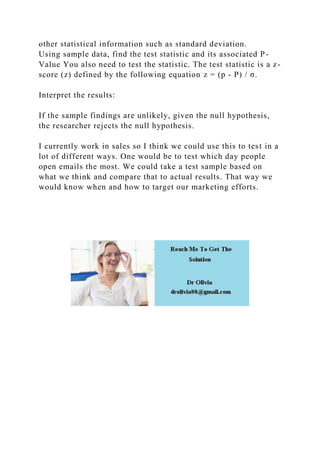Próximos SlideShares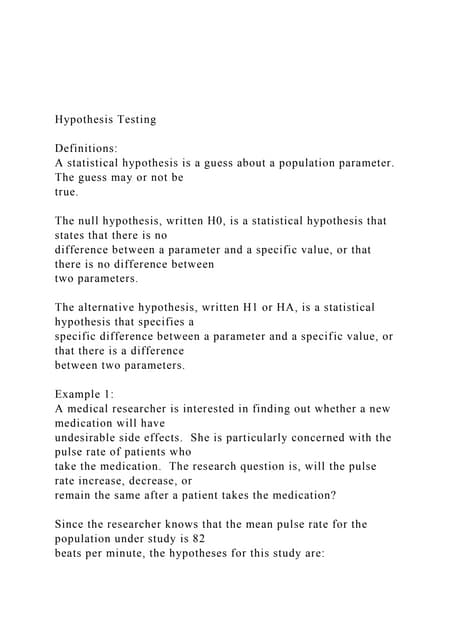Hypothesis Testing Definitions A statistical hypothesi.docx
Carregando em ... 3
1 de 5
Anúncio

### Question 1Discuss the process to perform hypothesis testin.docx

1. Question 1 Discuss the process to perform hypothesis testing problems about a population proportion using the z statistic. Give an example how you could use this process in your current position: I am a Property Manager which means I am like a landlord (I don’t own but work for owners). Discuss the process to define rejection and non-rejection zones, Give an example Please provide 130 words response to above question and example with reference if used Below 6 more questions are there, need 80 words response to each and an example, with reference if used. Chpt 9 pt. 2 1. A confidence interval is a range of values that is likely to contain an unknown population parameter. If you draw a random sample many times, a certain percentage of the confidence intervals will contain the population mean. This percentage is the confidence level. Most frequently, you'll use confidence intervals to bind the mean or standard deviation, but you can also obtain them for regression coefficients, proportions, rates of occurrence (Poisson), and for the differences between populations. Just as there is a common misconceptionof how to interpret P value, there's a common misconception of how to interpret confidence intervals. In this case, the confidence level is not the probability that a specific confidence interval contains the
2. population parameter. 2. Hypothesis testing is when we are looking for whether we can reject the null hypothesis or reject it. Confidence Interval tell us the range of possible values that correlate to the quantities. There is a close relationship between the two when comparing values. When a 95% confidence interval is considered we need to assess all the values in the interval and they are considered possible values. Values that are outside the interval are rejected as implausible. If the value specified by the null hypothesis is not in the interval than the null hypothesis can be rejected at the .5 level. So nothing the hypothesis testing will help us to determine the confidence intervals and what can be accepted or rejected. Chptr 9 pt. 1 3. Step 4 of the hypothesis process is to establish the decision rule by using alpha and the test statistic gathered in step 1 for critical values to be determined. The critical values are used at the end of the hypothesis decisioning step 7, in order to determine whether the null hypothesis is rejected or not. The possible outcomes of a study are divided into two groups: outcomes that reject the null hypothesis, and those that do not reject the null hypothesis. Statistical outcomes that result in the rejection of the null hypothesis lie in the rejection region. Outcomes that fail to result in the rejection of the null hypothesis lie in the non- rejection region. As such, when drawing a normal distribution curve, the mean is always in the middle at the highest point of the curve. Once the critical values are determined, they are placed in the tail of the curve to separate the non-rejection region from the means that do reject the hypothesis region. The rejection region is on the tail of both ends of the curve and falls beyond the critical values.
3. I work in a toy plant and want to test if the weight of the toy falls within 5 oz, which is the mean weight of the toy. My hypothesis is that the production is steadily producing the toys at an average weight of 5 oz. To test the hypothesis, I choose a random sample of 100 of those toys and find that the sample mean is 6 oz. Therefore, my hypothesis that the toys are all 5 oz that are produced on the line has rejected the null hypothesis. If however, the sample returns a sample mean of 5.3 oz, I choose to not reject the null hypothesis because in reality not all of the toys will weigh 5.2 oz and because the sample mean is considerably smaller, I do not have to worry. 4. The rejection and non-rejection zones are parts of hypothesis test. They are the areas of probability that tells you if your theory is true or probably not true. The area out the hypothesis test or that doesn't fall within the z score can be rejected. That means those values will not fall within your specified area. The area that is within the z score is the non-rejection region. An example of this would be say for instance a researcher wants to know the average household income. They come up with a hypothesis theory that the average income of 50,000. If the researcher takes random samples to get the sample mean and those samples are say 47,000 and 48,000 they might decide to accept the hypothesis. However, if the samples are much smaller closer to 35,000 they may decide to reject it which would be considered the rejection region. Chptr 9 pt. 3 5. According to the PowerPoint this is an eight step process: 1. Establish the hypothesis. 2. Determine the appropriate statistical test. 3. Set the value of α, the Type I error rate. 4. Establish the decision rule. 5. Gather the sample data. 6. Analyze the data. 7. Reach a statistical conclusion.
4. 8. Make a business decision. In my current position I could use this process to determine what types of product offering tend to be favored in different regions of the country. I work for a small electric vehicle manufacturer and we could use this data to make determinations about what products we offer & how successful we are with them in different regions. For example, we could take a sample population of the vehicles that we produce that have heaters installed in them. Once we have that data we can then use with data that tells which of the vehicles go where and at what times of the year. Do we sell most of our heater equipped vehicles into the Northeastern US in the months of July and August as customers begin prepare their facilities there for the winter months as compared to selling them into South Florida in March and April as the summer approaches. While this seems obvious to me it illustrates how this works. 6. There are several steps to hypothesis testing when using the z statistic. These include stating the hypothesis, formulating an analysis plan, analyzing any sample data and interpreting the results. To begin hypothesis testing you must first state the null hypothesis and an alternative hypothesis. Hypothesis must be stated in an exclusive way where if one is true the other is false. After that you must now formulate an analysis plan. To do this you must describe how to use sample data to either accept or reject the null hypothesis. It should also include 1. Significance level. 2. Test method. Using a z test to determine whether the population proportion differs from the observed sample. From there you use the sample data to test the p value as well as
5. other statistical information such as standard deviation. Using sample data, find the test statistic and its associated P- Value You also need to test the statistic. The test statistic is a z- score (z) defined by the following equation z = (p - P) / σ. Interpret the results: If the sample findings are unlikely, given the null hypothesis, the researcher rejects the null hypothesis. I currently work in sales so I think we could use this to test in a lot of different ways. One would be to test which day people open emails the most. We could take a test sample based on what we think and compare that to actual results. That way we would know when and how to target our marketing efforts.
Anúncio